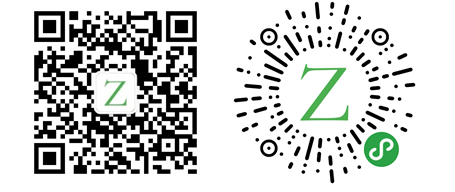# 走进 Rust：函数

Rust 大约 1042 字

## 命名规范

``````fn main() {
another_function(5, 6);
}

fn another_function(x: i32, y: i32) {
println!("The value of x is: {}", x);
println!("The value of y is: {}", y);
}``````

## Statements

``````fn main() {
let y = 6;
}``````

## Expressions

``````fn main() {
let x = 3;

let y = {
let x = 5;
x + 1;
x + 2
};
// y=7
println!("y={}", y);
}``````

## 函数返回值

``````fn five() -> i32 {
5
}

fn main() {
let x = five();

// The value of x is: 5
println!("The value of x is: {}", x);
}``````

``````fn main() {
let x = plus_one(5);

println!("The value of x is: {}", x);
}

fn plus_one(x: i32) -> i32 {
x + 1
}``````

————        END        ————

Give me a Star, Thanks:)

https://github.com/fendoudebb